login  home  contents  what's new  discussion  bug reports help  links  subscribe  changes  refresh  edit

  Date: Wed, Jul 4, 2018 at 5:45 AM
Subject: Factorization in XDPOLY ...


Since I never tried the ansatz (of Daniel Smertnig) and I needed something to warm up again (for programming in FriCAS) I did it now ...

## Factorization of non-commutative polynomials

in the free associative algebra XDPOLY using an ansatz

Idea: Daniel Smertnig, January 26, 2017

Test: Konrad Schrempf, Mit 2018-07-04 10:33

Definitions

fricas
--)read nc_ini03
ALPHABET := ['x, 'y, 'z];
Type: List(OrderedVariableList([x,y,z]))
fricas
OVL ==> OrderedVariableList(ALPHABET)
Type: Void
fricas
OFM ==> FreeMonoid(OVL)
Type: Void
fricas
F ==> Integer
Type: Void
fricas
G ==> Fraction(Polynomial(Integer))
Type: Void
fricas
XDP ==> XDPOLY(OVL, F)
Type: Void
fricas
YDP ==> XDPOLY(OVL, G)
Type: Void
fricas
--NCP ==> NCPOLY(OVL, F)
x := 'x::OFM;
fricas
y := 'y::OFM;
fricas
z := 'z::OFM;
fricas
OF ==> OutputForm
Type: Void

fricas
leftSubwords(p:XDP) : List(YDP) ==
lst_wrd : List(OFM) := []
for mon in support(p) repeat
wrd := 1$OFM for fct in factors(mon) repeat for i in 1 .. fct.exp repeat pos := position(wrd, lst_wrd)::NNI if zero?(pos) then lst_wrd := cons(wrd, lst_wrd) wrd := wrd*(fct.gen)::OFM lst_pol : List(YDP) := [] cnt_pol := #lst_wrd for wrd in lst_wrd repeat sym_tmp := (a[cnt_pol])::Symbol lst_pol := cons(sym_tmp*wrd::YDP, lst_pol) cnt_pol := (cnt_pol-1)::NNI lst_pol Function declaration leftSubwords : XDistributedPolynomial( OrderedVariableList([x,y,z]),Integer) -> List( XDistributedPolynomial(OrderedVariableList([x,y,z]),Fraction( Polynomial(Integer)))) has been added to workspace. Type: Void fricas rightSubwords(p:XDP) : List(YDP) == lst_wrd : List(OFM) := [] for mon in support(p) repeat wrd := 1$OFM
for fct in reverse(factors(mon)) repeat
for i in 1 .. fct.exp repeat
pos := position(wrd, lst_wrd)::NNI
if zero?(pos) then
lst_wrd := cons(wrd, lst_wrd)
wrd := (fct.gen)::OFM*wrd
lst_pol : List(YDP) := []
cnt_pol := #lst_wrd
for wrd in lst_wrd repeat
sym_tmp := (b[cnt_pol])::Symbol
lst_pol := cons(sym_tmp*wrd::YDP, lst_pol)
cnt_pol := (cnt_pol-1)::NNI
lst_pol
Function declaration rightSubwords : XDistributedPolynomial(
OrderedVariableList([x,y,z]),Integer) -> List(
XDistributedPolynomial(OrderedVariableList([x,y,z]),Fraction(
Polynomial(Integer)))) has been added to workspace.
Type: Void
fricas
factorizationPolynomial(p:XDP) : YDP ==
lsw := leftSubwords(p)
rsw := rightSubwords(p)
fp := 0\$YDP
for lw in lsw repeat
for rw in rsw repeat
fp := fp + lw*rw
fp
Function declaration factorizationPolynomial :
XDistributedPolynomial(OrderedVariableList([x,y,z]),Integer) ->
XDistributedPolynomial(OrderedVariableList([x,y,z]),Fraction(
Polynomial(Integer))) has been added to workspace.
Type: Void
fricas
factorizationEquations(p:XDP) : List(G) ==
lst_eqn : List(G) := []
fp := factorizationPolynomial(p)
for mon in support(fp) repeat
c_1 := coefficient(p, mon)
c_2 := coefficient(fp, mon)
lst_eqn := cons(c_2-c_1::G, lst_eqn)
for mon in support(p) repeat
if zero?(coefficient(fp, mon)) then
lst_eqn := []
break
lst_eqn
Function declaration factorizationEquations : XDistributedPolynomial
(OrderedVariableList([x,y,z]),Integer) -> List(Fraction(
Polynomial(Integer))) has been added to workspace.
Type: Void

Helper functions

Lift XDP over integers to YDP over rational functions and back

fricas
mapPoly(p:XDP):YDP ==
if reductum p = 0 then
else
Function declaration mapPoly : XDistributedPolynomial(
OrderedVariableList([x,y,z]),Integer) -> XDistributedPolynomial(
OrderedVariableList([x,y,z]),Fraction(Polynomial(Integer))) has
been added to workspace.
Type: Void
fricas
mapPoly2XDP(p:YDP):XDP ==
if reductum p = 0 then
else
Function declaration mapPoly2XDP : XDistributedPolynomial(
OrderedVariableList([x,y,z]),Fraction(Polynomial(Integer))) ->
XDistributedPolynomial(OrderedVariableList([x,y,z]),Integer) has
been added to workspace.
Type: Void
fricas
vars(p)==concat map(variables,coefficients(p))
Type: Void

Example 0:

fricas
p0 := factorizationEquations(x::XDP)
fricas
Compiling function leftSubwords with type XDistributedPolynomial(
OrderedVariableList([x,y,z]),Integer) -> List(
XDistributedPolynomial(OrderedVariableList([x,y,z]),Fraction(
Polynomial(Integer))))
fricas
Compiling function rightSubwords with type XDistributedPolynomial(
OrderedVariableList([x,y,z]),Integer) -> List(
XDistributedPolynomial(OrderedVariableList([x,y,z]),Fraction(
Polynomial(Integer))))
fricas
Compiling function factorizationPolynomial with type
XDistributedPolynomial(OrderedVariableList([x,y,z]),Integer) ->
XDistributedPolynomial(OrderedVariableList([x,y,z]),Fraction(
Polynomial(Integer)))
fricas
Compiling function factorizationEquations with type
XDistributedPolynomial(OrderedVariableList([x,y,z]),Integer) ->
List(Fraction(Polynomial(Integer)))
fricas
Compiling function G742 with type Integer -> Boolean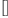(1)
Type: List(Fraction(Polynomial(Integer)))
fricas
solve(p0)
>> Error detected within library code:
No identity element for reduce of empty list using operation
setUnion

shows that x is irreducible ;-).

Well for non-trivial polynomials solve does not work. One could try Groebner- Shirshov bases, etc.

In principle it should work with general base rings, for example the integers. But I do not know the capabilities of solve. Anyway, I hope that it could be useful within XDPOLY (at least for small polynomials, because the number of non-linear equations is increasing exponentially).

The file in the attachment is meant to put on github for discussions.

https://github.com/billpage/ncpoly

Example 1:

fricas
p_1 : XDP := x*(1-y*x);
fricas
l1 := reduce(+,leftSubwords(p_1))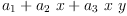(2)
Type: XDistributedPolynomial(OrderedVariableList([x,y,z]),Fraction(Polynomial(Integer)))
fricas
r1 := reduce(+,rightSubwords(p_1))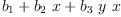(3)
Type: XDistributedPolynomial(OrderedVariableList([x,y,z]),Fraction(Polynomial(Integer)))
fricas
e1 := factorizationEquations(p_1)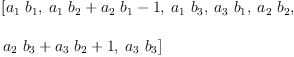(4)
Type: List(Fraction(Polynomial(Integer)))
fricas
concat(vars l1, rest vars r1)
fricas
Compiling function vars with type XDistributedPolynomial(
OrderedVariableList([x,y,z]),Fraction(Polynomial(Integer))) ->
List(Symbol)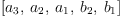(5)
Type: List(Symbol)
fricas
s1:=solve(e1,concat(vars l1, rest vars r1))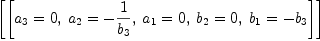(6)
Type: List(List(Equation(Fraction(Polynomial(Integer)))))
fricas
fl1:=map((x:G):G+->eval(x,s1.1),l1)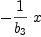(7)
Type: XDistributedPolynomial(OrderedVariableList([x,y,z]),Fraction(Polynomial(Integer)))
fricas
fr1:=map((x:G):G+->eval(x,s1.1),r1)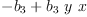(8)
Type: XDistributedPolynomial(OrderedVariableList([x,y,z]),Fraction(Polynomial(Integer)))
fricas
fl1*fr1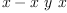(9)
Type: XDistributedPolynomial(OrderedVariableList([x,y,z]),Fraction(Polynomial(Integer)))

Example 2:

fricas
p_2 : XDP := x*y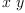(10)
fricas
l2 := reduce(+,leftSubwords(p_2))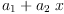(11)
Type: XDistributedPolynomial(OrderedVariableList([x,y,z]),Fraction(Polynomial(Integer)))
fricas
r2 := reduce(+,rightSubwords(p_2))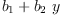(12)
Type: XDistributedPolynomial(OrderedVariableList([x,y,z]),Fraction(Polynomial(Integer)))
fricas
e2 := factorizationEquations(p_2)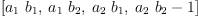(13)
Type: List(Fraction(Polynomial(Integer)))
fricas
s2:=solve(e2,concat(vars l2, rest vars r2))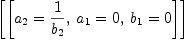(14)
Type: List(List(Equation(Fraction(Polynomial(Integer)))))
fricas
fl2:=map((x:G):G+->eval(x,s2.1),l2)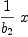(15)
Type: XDistributedPolynomial(OrderedVariableList([x,y,z]),Fraction(Polynomial(Integer)))
fricas
fr2:=map((x:G):G+->eval(x,s2.1),r2)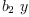(16)
Type: XDistributedPolynomial(OrderedVariableList([x,y,z]),Fraction(Polynomial(Integer)))
fricas
fl2*fr2(17)
Type: XDistributedPolynomial(OrderedVariableList([x,y,z]),Fraction(Polynomial(Integer)))

Example 3:

fricas
p_3 : XDP := (x-y)*(x+y)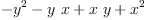(18)
fricas
l3 := reduce(+,leftSubwords(p_3))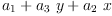(19)
Type: XDistributedPolynomial(OrderedVariableList([x,y,z]),Fraction(Polynomial(Integer)))
fricas
r3 := reduce(+,rightSubwords(p_3))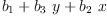(20)
Type: XDistributedPolynomial(OrderedVariableList([x,y,z]),Fraction(Polynomial(Integer)))
fricas
e3 := factorizationEquations(p_3)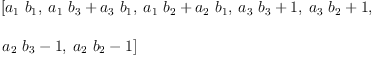(21)
Type: List(Fraction(Polynomial(Integer)))
fricas
s3:=solve(e3,concat(vars l3, rest vars r3))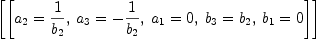(22)
Type: List(List(Equation(Fraction(Polynomial(Integer)))))
fricas
fl3:=map((x:G):G+->eval(x,s3.1),l3)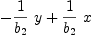(23)
Type: XDistributedPolynomial(OrderedVariableList([x,y,z]),Fraction(Polynomial(Integer)))
fricas
fr3:=map((x:G):G+->eval(x,s3.1),r3)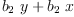(24)
Type: XDistributedPolynomial(OrderedVariableList([x,y,z]),Fraction(Polynomial(Integer)))
fricas
fl3*fr3(25)
Type: XDistributedPolynomial(OrderedVariableList([x,y,z]),Fraction(Polynomial(Integer)))

In order to obtain a solution we must choose (to omit) one variable that is necessarily not 0 since it is going to appear in the denominator of a coefficient in the result.

Although this solution might be a bit "heavy" [groebnerFactorize]? expresses the solution as a union of ideals. In each ideal those variables that are necessarily zero will appear as bases containing only one variable. The remaining variables are "significant" and we can choose any of these as parameters.

fricas
param(e) == first variables first remove((x:G):Boolean+->#variables(x)<2, e)
Type: Void

Example 4:

fricas
p_4 : XDP := (x-y^2)*(x+z^2)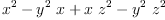(26)
fricas
l4 := reduce(+,leftSubwords(p_4))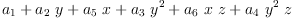(27)
Type: XDistributedPolynomial(OrderedVariableList([x,y,z]),Fraction(Polynomial(Integer)))
fricas
r4 := reduce(+,rightSubwords(p_4))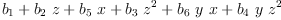(28)
Type: XDistributedPolynomial(OrderedVariableList([x,y,z]),Fraction(Polynomial(Integer)))
fricas
e4 := factorizationEquations(p_4)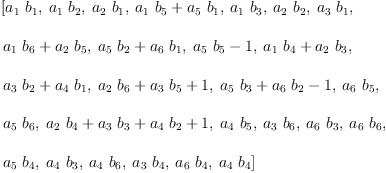(29)
Type: List(Fraction(Polynomial(Integer)))
fricas
groebnerFactorize e4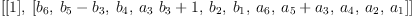(30)
Type: List(List(Polynomial(Integer)))
fricas
e4a := last %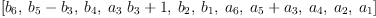(31)
Type: List(Polynomial(Integer))
fricas
param(e4a)
fricas
Compiling function param with type List(Polynomial(Integer)) ->
Symbol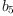(32)
Type: Symbol
fricas
)set output tex off

fricas
)set output algebra on
--s4:=solve(e4,concat(vars l4, rest vars r4))
s4:=solve(e4a,remove(param(e4a), concat(vars l4,vars r4)) )
(53)
[
1        1
[a  = 0, a  = 0, a  = - --, a  = --, a  = 0, a  = 0, b  = 0, b  = 0,
4       6       3     b    5   b    2       1       4       6
5        5
b  = b , b  = 0, b  = 0]
3    5   2       1
]
Type: List(List(Equation(Fraction(Polynomial(Integer)))))
fricas
)set output tex on

fricas
)set output algebra off
fl4:=map((x:G):G+->eval(x,s4.1),l4)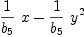(33)
Type: XDistributedPolynomial(OrderedVariableList([x,y,z]),Fraction(Polynomial(Integer)))
fricas
fr4:=map((x:G):G+->eval(x,s4.1),r4)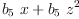(34)
Type: XDistributedPolynomial(OrderedVariableList([x,y,z]),Fraction(Polynomial(Integer)))
fricas
fl4*fr4(35)
Type: XDistributedPolynomial(OrderedVariableList([x,y,z]),Fraction(Polynomial(Integer)))
fricas
test(mapPoly p_4 = fl4*fr4)
fricas
Compiling function mapPoly with type XDistributedPolynomial(
OrderedVariableList([x,y,z]),Integer) -> XDistributedPolynomial(
OrderedVariableList([x,y,z]),Fraction(Polynomial(Integer)))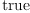(36)
Type: Boolean

Example 5:

fricas
p_5 : XDP := (x*y*z+y*x*z)*(z*x*y+z*y*x)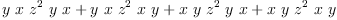(37)
fricas
l5 := reduce(+,leftSubwords(p_5))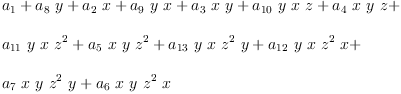(38)
Type: XDistributedPolynomial(OrderedVariableList([x,y,z]),Fraction(Polynomial(Integer)))
fricas
r5 := reduce(+,rightSubwords(p_5))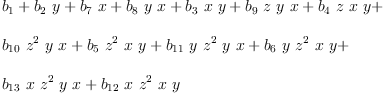(39)
Type: XDistributedPolynomial(OrderedVariableList([x,y,z]),Fraction(Polynomial(Integer)))
fricas
e5 := factorizationEquations(p_5)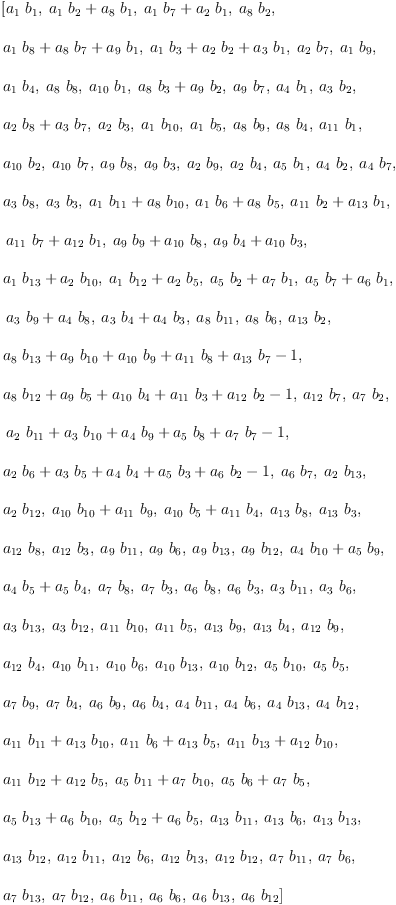(40)
Type: List(Fraction(Polynomial(Integer)))
fricas
)set output tex off

fricas
)set output algebra on
-- look for a solution
for i in 1..#coefficients r5 repeat
s5 := solve(e5,concat(vars l5, remove(b[i],vars r5)))
#s5.1>0 => break
Type: Void
fricas
s5
(63)
[
1         1
[a  = 0, a  = 0, a   = 0, a   = 0, a  = --, a   = --, a  = 0, a   = 0,
6       7       12       13       5   b    11   b    4       10
3         3
a  = 0, a  = 0, a  = 0, a  = 0, a  = 0, b   = 0, b   = 0, b  = 0,
3       9       2       8       1       12       13       6
b   = 0, b  = 0, b   = 0, b  = 0, b  = 0, b  = b , b  = 0, b  = 0,
11       5       10       4       9       8    3   7       2
b  = 0]
1
]
Type: List(List(Equation(Fraction(Polynomial(Integer)))))
fricas
)set output tex on

fricas
)set output algebra off
fl5:=map((x:G):G+->eval(x,s5.1),l5)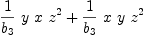(41)
Type: XDistributedPolynomial(OrderedVariableList([x,y,z]),Fraction(Polynomial(Integer)))
fricas
fr5:=map((x:G):G+->eval(x,s5.1),r5)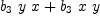(42)
Type: XDistributedPolynomial(OrderedVariableList([x,y,z]),Fraction(Polynomial(Integer)))
fricas
fl5*fr5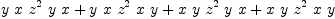(43)
Type: XDistributedPolynomial(OrderedVariableList([x,y,z]),Fraction(Polynomial(Integer)))
fricas
test(mapPoly p_5 = fl5*fr5)(44)
Type: Boolean

Example 6:

We need the solution of the factorization equations to associate a unique value with each variable. If the result includes any implicit solutions, i.e. equations whose lefthand side is not just a symbol then solve was not able to find a full solution. In this case the expression is irreducible over the base domain.

fricas
p_6 : XDP := 2 - x^2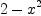(45)
fricas
l6 := reduce(+,leftSubwords(p_6))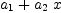(46)
Type: XDistributedPolynomial(OrderedVariableList([x,y,z]),Fraction(Polynomial(Integer)))
fricas
r6 := reduce(+,rightSubwords(p_6))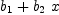(47)
Type: XDistributedPolynomial(OrderedVariableList([x,y,z]),Fraction(Polynomial(Integer)))
fricas
e6 := factorizationEquations(p_6)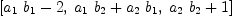(48)
Type: List(Fraction(Polynomial(Integer)))
fricas
-- look for a solution
for i in 1..#coefficients r6 repeat
s6 := solve(e6,concat(vars l6, remove(b[i],vars r6)))
#s6.1>0 => break
Type: Void
fricas
s6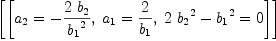(49)
Type: List(List(Equation(Fraction(Polynomial(Integer)))))

Test for irreducibility.

fricas
map(x+->retractIfCan(lhs x)@Union(Symbol,"failed") case Symbol, s6.1)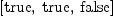(50)
Type: List(Boolean)
fricas
if reduce(_and, %) then
fl6:=map((x:G):G+->eval(x,s6.1),l6)
fr6:=map((x:G):G+->eval(x,s6.1),r6)
fl6*fr6
test(mapPoly p_6 = fl6*fr6)
else
"irreducible"::"irreducible"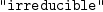(51)
Type: irreducible

 Subject:   Be Bold !! ( 14 subscribers )